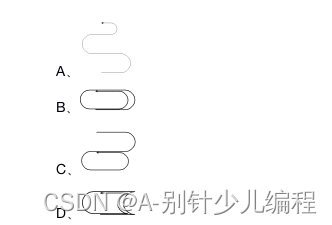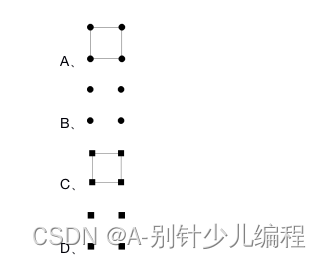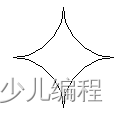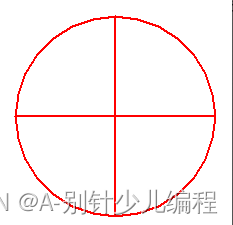### 2020年6月电子学会Python等级考试试卷（一级）考题解析1. 以下哪种输入结果不可能得到以下反馈： 重要的事情说三遍：安全第一！安全第一！安全第一！（ ）

A、print(“重要事情说三遍：”+”安全第一！”*3)

B、print(“重要事情说三遍：”+”安全第一！“+”安全第一！”*2)

C、print(“重要事情说三遍：”+”安全第一！”+”安全第一！”+”安全第一！”)

D、print(“重要事情说三遍：”+”安全第一！”/3)

2. 运行下列程序后，绘制出以下哪个图形？（ ）

import turtle

turtle.pensize(3)

turtle.forward(150)

turtle.circle(50,180)

turtle.forward(180)

turtle.circle(48,180)

turtle.forward(150)

turtle.circle(45,180)

turtle.forward(120)

turtle.done()3. 执行print(1 + 2 * 2+ 6 / 3) 的结果为?（ ）

A、4

B、7

C、4.0

D、7.0

4. 已知变量x=2，语句print(“x=”,x)的作用是？（ ）

A、在屏幕上输出x=x

B、在屏幕上输出2=2

C、在屏幕上输出x=2

D、在屏幕上输出“x=”2

5.

import turtle

t1=turtle.Turtle(‘turtle’)

t2=turtle.Turtle(‘turtle’)

t3=turtle.Turtle(‘turtle’)

t4=turtle.Turtle(‘turtle’)

t1.forward(50)

t2.forward(100)

t3.forward(150)

t4.forward(200)

A、0

B、1

C、4

D、5

6. print(24%5),运算结果是？（ ）

A、1

B、2

C、3

D、4

7. 下面哪个指令不可以让海龟回到坐标（0，0）点？（ ）

A、turtle.goto(0,0)

B、turtle.home()

C、turtle.setposition(0,0)

D、turtle.set(0,0)

8.

a=30

b=5

print(a/b)

A、6

B、30/5

C、6.00

D、6.0

9. print(46//8)的结果是？（   ）

A、5

B、6

C、5.7

D、5.75

10. Python启动后显示的提示符是？（ ）

A、c:\>

B、>>>

C、—

D、%%%

11. 下列代码不能画出直径为10的点的是?（ ）

A、turtle.pensize(10)

turtle.pendown()

B、turtle.dot(10)

C、turtle.begin_fill()

turtle.circle(5)

turtle.end_fill()

D、turtle.begin_fill()

turtle.circle(10)

turtle.end_fill()

12. 已知变量a=5，b=6，执行语句a*=a+b后，变量a的值为：（ ）

A、11

B、30

C、31

D、55

13. 如果a=23，b=10，那么print（a%b）的结果是？（ ）

A、2

B、3

C、23

D、2.3

14. 下列表达式的值为True的是？（ ）

A、’a’>’b’

B、2>3

C、’A’>’a’

D、’3′>’2′

15. 已知x=5，y=6，则表达式not(x!=y)的值为：（ ）

A、True

B、False

C、5

D、6

16. 执行下面程序后，以下哪个图形是正确的？（ ）

import turtle

turtle.shape(‘square’)

turtle.home()

turtle.dot()

turtle.stamp()

turtle.forward(100)

turtle.stamp()

turtle.forward(100)

turtle.left(90)

turtle.stamp()

turtle.forward(100)

turtle.left(90)

turtle.stamp()

turtle.forward(100)17. 输出如下古诗，请问哪句是正确的？（ ）

A、print(

‘闻道梅花坼晓风，雪堆遍满四山中。

何方可化身千亿，一树梅花一放翁。’)

B、print(‘闻道梅花坼晓风，雪堆遍满四山中。’

‘何方可化身千亿，一树梅花一放翁。’)

C、print(”’闻道梅花坼晓风，雪堆遍满四山中。

D、print(“闻道梅花坼晓风，雪堆遍满四山中。”\n

“何方可化身千亿，一树梅花一放翁。”)

18.

a=123

print(a%100%10)

A、1

B、2

C、3

D、1.23

19.turtle.circle(50,90)

turtle.circle(-50,-90)

turtle.circle(50,90)

turtle.circle(-50,-90)

turtle.circle(50,90)

turtle.circle(-50,-90)

turtle.circle(-50,-90)

turtle.circle(50,90)

turtle.circle(50,90)

turtle.circle(50,90)

turtle.circle(-50,-90)

turtle.circle(-50,-90)

turtle.circle(-50,-90)

turtle.circle(50,90)

turtle.circle(50,90)

turtle.circle(-50,-90)

20. 下面描述中，不符合Python语言特点的是：（ ）

A、Python是一门面向对象的编程语言

B、Python程序通过编译后执行

C、Python支持函数编程

D、Python支持多个操作系统

21. 下列哪个不是Python的保留字？（ ）

A、if

B、or

C、do

D、for

22. 执行下列语句后的显示结果是什么？（ ）

b = 2 * a / 4

a = 1

print(a,b)

A、1 , 0.5

B、1 , 0

C、报错

D、0 , 1

23. a=5 print(‘a+4′) 以上两段代码的结果是？（ ）

A、9

B、’a+4‘

C、无结果，出错

D、a+4

24. 以下选项中，Python语言中代码注释使用的符号是？（ ）

A、/… …/

B、！

C、#

D、//

25.

import turtle

turtle.goto(0,0)

turtle.goto(0,100)

turtle.goto(100,100)

turtle.goto(100,0)

turtle.goto(0,0)

A、水平向左

B、水平向右

C、垂直向上

D、垂直向下

26.

print( “a”, end=” ” )

print(“b”, end=” ” )

27.

print()函数不可以在屏幕上打印出空行。

28. Turtle库中，使用circle(20)命令，指的是画出以画布正中央为圆心，半径为20的圆形。

29. 在IDLE编辑器中，Python代码的字体和字号可以根据需要自行设置，方便大家的使用。

30.

Abc、aBc、abC是三个不同的变量。

31.

one,two,three=’1′,3,5; print(one+two+three)运行结果是9。

32. a*=b，就是a=b*b。

33.

Python除了用自带的IDLE进行编程外还可以用其他编程环境进行程序编写，比如JupyterNotebook。

34. turtle.circle(50,steps=5)命令可以画一个五角星。

35. is和input都是关键字，不能随意使用。

36.

（1）画出如下的图形，注：直线部分是由两个步长为200的线段垂直相交组成，圆的直径为200；

（2）图形的中心位置为画布中心；

（3）画笔宽度为2，颜色为红色。（1）正确导入turtle库;（1分）

（2）有前进命令；（1分）

（3）有画圆命令；（1分）

（4）有转直角弯命令；（1分）

（5）图形中心位置为画布中心；（1分）

（6）颜色和画笔宽度均达要求，各1分；（2分）

（7）运行正常；（1分）

（8）完成任务。（2分）

import turtle

turtle.pencolor(“red”)

turtle.pensize(2)

turtle.forward(-100)

turtle.forward(200)

turtle.left(90)

turtle.circle(100,450)

turtle.left(90)

turtle.forward(200)

#turtle.hideturtle()

turtle.done()

37.

（1）程序开始运行后，会有提示字符串：“请输入奶牛的头数：”，完成奶牛头数的输入；（可以直接赋值提示字符串到程序中，提示字符串包括冒号，但不包括双引号）

（2）程序会根据输入的奶牛头数计算出总共产出的牛奶的重量，并将结果进行修饰然后输出。示例：如果输入奶牛的头数为10，则输出“10 头奶牛7天可以产 1400 千克的牛奶

（1）有输入语句并且有清楚的提示字符串；（2分）

（2）有输出语句并且按照样例输出；（2分）

（3）有类型转化语句；（2分）

（4）有计算语句；（2分）

（5）程序符合题目要求。（2分）

1、参考程序仅供参考，学生可以有多个解决方法。如果学生使用格式输出的方式，只要结果符合要求即为正确；

2、建议将每一个考生的程序最少运行测试一次。

3、参考程序如下：

N = input(“请输入奶牛的头数：”)

N = eval(N)

milk = N*20*7

print(N,”头奶牛7天可以产”,milk,”千克的牛奶”)

N = input(“请输入奶牛的头数：”)

N = int(N)

milk = N*20*7

print(N,”头奶牛7天可以产”,milk,”千克的牛奶”)

N =int(input(“请输入奶牛的头数：”))

milk = N*20*7

print(N,”头奶牛7天可以产”,milk,”千克的牛奶”)

N = input(“请输入奶牛的头数：”)

N = int(N)

milk = N*20*7

print(str(N)+”头奶牛7天可以产”+str(milk)+”千克的牛奶”)

N = input(“请输入奶牛的头数：”)

N = int(N)

milk = N*20*7

print(“{}头奶牛7天可以产{}千克的牛奶”.format(N,milk))

N = input(“请输入奶牛的头数：”)

N = int(N)

milk = N*20*7

print(“%d头奶牛7天可以产%d千克的牛奶”%(N,milk))

38.

Python一级 面试题

1. 本题不需要作答；

2. 请考生进入面试间等待叫号进行面试，由主考官提问问题，考生回答。